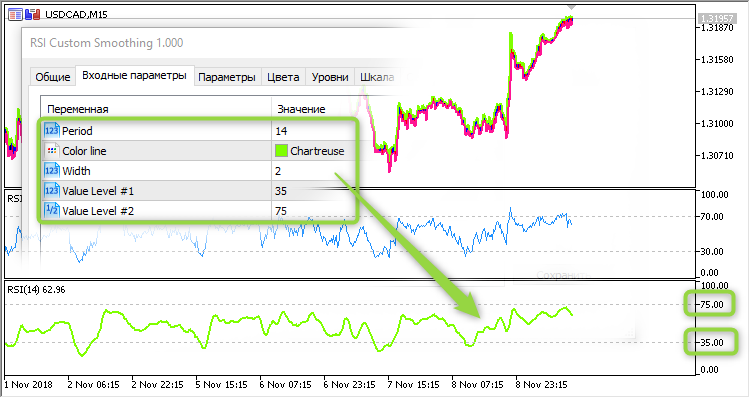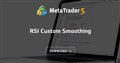# iRSIOnArray for MT5 indi?898

I want to move an old indicator to MQL 5 and therefor need iRSIOnArray equivalent. Searched, but couldn't find anything so far.

Would someone be so kind to point me to an implementation?38376

lippmaje:

I want to move an old indicator to MQL 5 and therefor need iRSIOnArray equivalent. Searched, but couldn't find anything so far.

Would someone be so kind to point me to an implementation?

Lazy ? ;-)898

Alain Verleyen:
Lazy ? ;-)

Ok, I took this as sort of hint and it seems there's some git hub code that could be used.

For anyone interested here's the link: https://github.com/Shmuma/mt-tools/blob/master/mt5/IndicatorsOnArray.mqh38376

lippmaje:

Ok, I took this as sort of hint and it seems there's some git hub code that could be used.

For anyone interested here's the link: https://github.com/Shmuma/mt-tools/blob/master/mt5/IndicatorsOnArray.mqh

:-D

I hope you don't have to use this implementation with a long period and a buffer as it will be very inefficient.

EDIT: And in my opinion this is a bug or at least leads to an not standard RSI :

```   if (neg > 1e-5)
rsi = 100.0 - 100.0 / (1 + pos / neg);```898

Alain Verleyen:

:-D

I hope you don't have to use this implementation with a long period and a buffer as it will be very inefficient.

Is there something more efficient or is it possible to pass an 'array' handle to iRSI?38376

lippmaje:
Is there something more efficient or is it possible to pass an 'array' handle to iRSI?

You can look at XXXXOnBuffer() functions in MovingAverages.mqh (provided with MT5) and code RSI in a similar way.

Hopefully one day I will be allowed to share my codes :-)898

Will do so and post something here when I got my head around this.

Thank you.

---

And this looks like a bug, too, it should be nsum:

```   pos = psum / period;
neg = psum / period;```38376

lippmaje:

Will do so and post something here when I got my head around this.

Thank you.

---

And this looks like a bug, too, it should be nsum:

Yeah, 2 bugs in such a simple code. I am suggesting you to use the RSI.mq5 you will found in Indicators\Examples as a base.Moderator
164327

lippmaje :

I want to move an old indicator to MQL 5 and therefor need iRSIOnArray equivalent. Searched, but couldn't find anything so far.

Would someone be so kind to point me to an implementation?

Indicator example:

RSI Custom Smoothing

The following settings are now available as input parameters: color of the indicator main line width of the indicator main line values of the two indicator levels What is the purpose of the above updates: now, indicator levels and colors can be managed from within the Expert Advisor. This feature is especially useful during visual testing. For example, non-standard levels are set in the EA: 35 and 75. When using the RSI Custom Smoothing in the visual testing mode, the levels of 35 and 75 will be displayed on the chart. Indicator buffer smoothing has been added. Smoothing is performed though a Simple averaging method with the period of 6. An example of creating an indicator handle in the EA: //--- create handle of the indicator iRSI handle_iCustom= iCustom (m_symbol.Name(), Period (), 'RSI Custom Smoothing ',Inp_RSI_Period, Inp_RSI_Color,Inp_RSI_Width,Inp_RSI_Level1,Inp_RSI_Level2); //--- if the handle is not created if (handle_iCustom== INVALID_HANDLE ). {. //--- tell about the...

CodeBase | 2019.01.02 14:31 |  Vladimir Karputov | Indicators | MetaTrader 5RSI Custom Smoothing
• www.mql5.com
What is the purpose of the above updates: now, indicator levels and colors can be managed from within the Expert Advisor. This feature is especially useful during visual testing. For example, non-standard levels are set in the EA: 35 and 75. When using the RSI Custom Smoothing...898

Thanks Vladimir, I've put everything together into a neat iRSIOnArray look-alike for MT5. Should work like the original:

```double iRSIOnArray_(double &array[],int total,int period,int shift)
{
if(total==0)
total=ArraySize(array);
int stop=total-shift;
if(period<=1 || shift<0 || stop<=period)
return 0;
bool isSeries=ArrayGetAsSeries(array);
if(isSeries)
ArraySetAsSeries(array,false);
int i;
double SumP=0;
double SumN=0;
for(i=1; i<=period; i++)
{
double diff=array[i]-array[i-1];
if(diff>0)
SumP+=diff;
else
SumN+=-diff;
}
double AvgP=SumP/period;
double AvgN=SumN/period;
for(; i<stop; i++)
{
double diff=array[i]-array[i-1];
AvgP=(AvgP*(period-1)+(diff>0?diff:0))/period;
AvgN=(AvgN*(period-1)+(diff<0?-diff:0))/period;
}
double rsi;
if(AvgN==0.0)
{
rsi=(AvgP==0.0 ? 50.0 : 100.0);
}
else
{
rsi=100.0-(100.0/(1.0+AvgP/AvgN));
}
if(isSeries)
ArraySetAsSeries(array,true);
return rsi;
}```